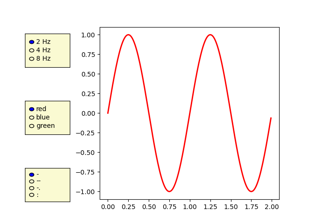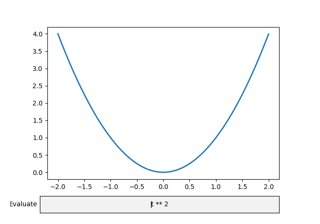# matplotlib.pyplot.draw#

matplotlib.pyplot.draw()[source]#

Redraw the current figure.

This is used to update a figure that has been altered, but not automatically re-drawn. If interactive mode is on (via `ion()`), this should be only rarely needed, but there may be ways to modify the state of a figure without marking it as "stale". Please report these cases as bugs.

This is equivalent to calling `fig.canvas.draw_idle()`, where `fig` is the current figure.

## Examples using `matplotlib.pyplot.draw`#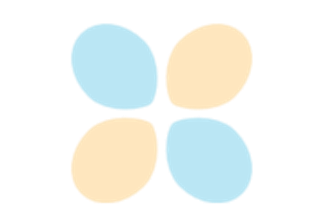Interactive functions

Interactive functionsRotating a 3D plot

Rotating a 3D plot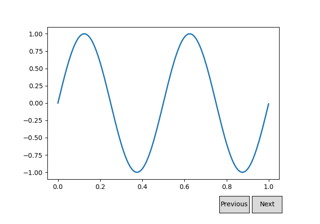Buttons

Buttons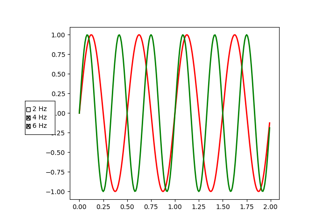Check Buttons

Check Buttons